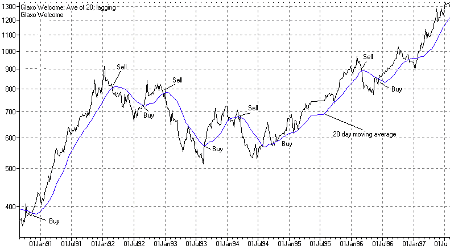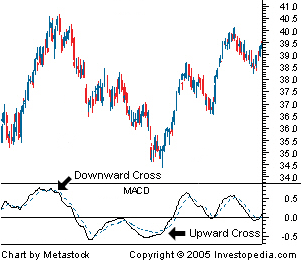### Would you like to switch to our mobile app?

Chapter 25 — Moving Averages
Font type: Sans-Serif
Font size: Large

## Moving Averages

One of the most popular and simple forms of technical analysis is following the trends of moving averages. They provide a way to smooth out the price fluctuations in a stock price and help you find a clear picture of upcoming price trends.

Average Share Price

To understand the concept of a moving average, it is important to know how an average is calculated. A simple average is calculated by adding up the closing price of a share for a number of time periods and dividing that total by the number of time periods. For example, the seven-day average would be calculated by taking seven days’ worth of closing prices, adding them together, and dividing the total by seven.Adding the prices together gives a total of 82.12. Dividing that number by seven gives the seven day average of 11.73. This is useful for understanding how the average is calculated, but it will not tell you anything about a price trend. For that, you need a moving average.

Moving Average

There are two variations of the moving average. The simple moving average and the weighted moving average. The only difference is that the weighted moving average puts a higher weight on more recent prices. This method is used with the assumption that more recent price data will have a larger impact on the future movement of the stock.

To get a moving average, you start by using the simple average from the first seven days of price information. We have already calculated the first point for the moving average. It is 11.73. To find the next point, we simply shift our time period to the next day.

For example, from the table, to find our second point for the moving average, we will use days 2 through 8. Days 3 through 9 for the third point, and so on. From these 10 days of pricing data, we will come up with four points for our moving average:When you graph the points of your moving average, you will come up with a graph that shows how the stock’s average price has moved over time. The higher number of days you use for your moving average, the “smoother” the moving average will appear. The fewer number of days you use for your average, the more “jerky” the moving average will be. Some technical analysis prefer to use the longer time periods because they will not get as many false readings, but others prefer to use more short-term averages because they believe it allows them to see a trend faster.

Interpreting Moving Averages

The simplest way to interpret a moving average is to see which direction the average is moving and treat it as a trend. However, this is not useful because you can find the same information by looking at the price. What most technical analysts do with a moving average is combine it with pricing data. The results are relatively simple to read: if the stock’s price moves above the moving average, it is a signal to buy. If the stock’s price moves below the moving average, it is a signal to sell. You may not capture the full movement of the trend prices, but you will always be on the correct side of the market.There are also more complex ways to analyze moving averages. One way is the Moving Average Convergence Divergence (MACD) model. This model compares the difference between a 26-day weighted moving average and a 12-day weighted moving average. This difference is called the MACD. A 9-day weighted average of the MACD is used as a “signal line” to determine whether to buy or sell.

The simplest way to interpret this signal line is to treat it the same way as you would the moving average: When the MACD “crosses over” and goes below its moving average, it is a signal to sell (downward cross). When the MACD crosses above the moving average, it is a signal to buy (upward cross). Some traders also consider a MACD movement above the zero line to be a buy signal (upward momentum), and a movement below the zero line to be a sell signal (downward momentum).